# Extension theorems (in analytic geometry)

Statements on the continuation (extension) of functions, sections of analytic sheaves, analytic sheaves, analytic subsets, holomorphic and meromorphic mappings, from the complement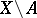in an analytic spaceof a set(as a rule, also analytic) to the whole space. Two theorems of B. Riemann form the classical results concerning continuation of functions.
Riemann's first theorem states that every analytic function on, whereis a normal complex space andan analytic subspace of codimension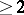, can be continued to an analytic function on. Riemann's second theorem states that every analytic functiononthat is locally bounded on, whereis a nowhere-dense analytic subset in a normal complex space, can be continued to an analytic function on. There are generalizations of these theorems to arbitrary complex spaces, as well as to sections of coherent analytic sheaves (cf. Local cohomology).
Important results concerning extension of analytic subsets are the theorems of Remmert–Stein–Shiffman and Bishop. The Remmert–Stein–Shiffman theorem states that every pure-dimensional complex-analytic subset in, whereis a complex-analytic space anda closed subset having zero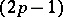-dimensional Hausdorff measure, can be extended to a pure-dimensional complex-analytic subset in. Bishop's theorem states that every pure-dimensional complex-analytic subsetin, whereis a complex-analytic space andis a complex-analytic subset, can be extended to a pure-dimensional complex-analytic subset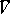inifhas locally finite volume in some neighbourhoodofin.
There are criteria for extendability of analytic mappings, generalizing the classical Picard theorem. E.g., every analytic mapping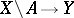, whereis a complex manifold,is an analytic nowhere-dense set andis a hyperbolic compact complex manifold, can be extended to an analytic mapping. Every analytic mappingthat is not everywhere-degenerate, whereis a complex manifold,is an analytic subset andis a compact complex manifold with negative first Chern class, can be extended to a meromorphic mapping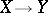.